## 11. Coulomb potential.

If a particle P with mass m is attracted by a body S at the origin O with a force inversely proportional to the square of its distance x (x > 0) from S, its potential energy is expressed by a function having form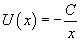where C is a positive proportionality constant depending on P, S and on medium between them. In this case the potential well has an outline like the following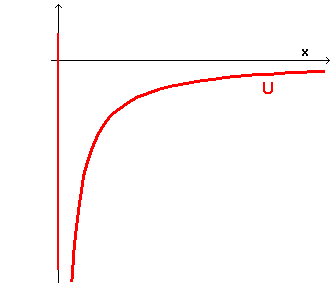If the total energy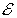of the particle is negative, the graph of energy is like this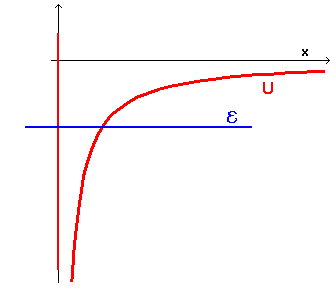The Schrödinger equation in this case is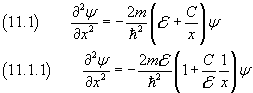If we let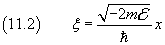we have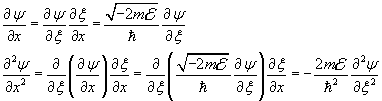and after, from (11.1.1),To simplify the notation we let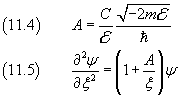x→∞ ⇒ ψ→0 , so we may assume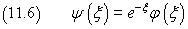where φ is a function to determine later, with a singularity for ξ=0 but finite for every other ξ.

By differentiating twice the ψ(x) in (11.6) with respect to ξ we have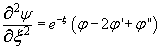and this expression of the second derivative in (11.5) gives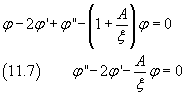Now, like in the previous section, we assume that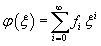and therefore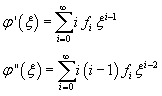By using these values in (11.7) we obtain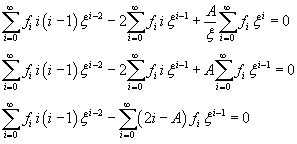Since φ is not defined for x=0, we do not consider the terms of the expansion with negative exponents of ξ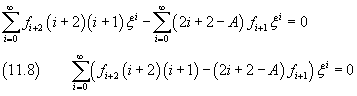The sum in (11.8) is zero only if all the coefficients of the powers of ξ are zero: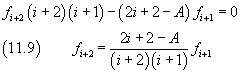Like in the previous section, we have obtained a recursive relationship between the coefficients of the series expansion. Furthermore, the expansion reduces to a polynomial, and therefore is finite for every ξ, if a not zero coefficient is followed by a coefficient equal to zero. Let k be the index of the last not zero coefficient. From (11.9) we obtain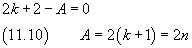that is A must be a not zero even natural number (in (11.10) n represents a natural number ≥ 1).

By recovering the definition of A from (11.4), from (11.10) we obtain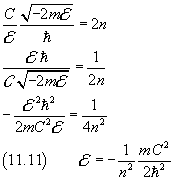Once more we reach the conclusion that the energy of a particle can have only discrete, quantized, values. The minimum value is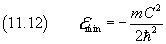If the particle is an electron in the field of a proton, we have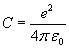and, from (11.1),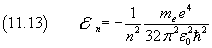The equation (11.13) coincides with that obtained by N. Bohr to explain the hydrogen spectrum, under the hypothesis that the electron has circular orbits such that its angular momentum is an integer multiple of the reduced Planck constant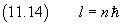In fact, if r is the orbital radius and v the electron speed, from (11.14)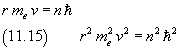Coulomb's force is a centripetal force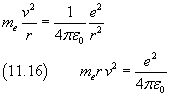By dividing the equation (11.16) by the equation (11.15) we obtain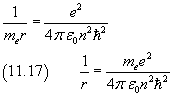The total energy of an electron with circular orbit in the field of a proton is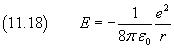Finally, from (11.17) and (11.18) we obtain (11.13)

In conclusion, the Schrödinger equation provides a general theoretical foundation to explain results previously obtained on the basis of ad hoc hypotheses, like these of Bohr, assumed to justify specific experimental data.